# AN131 - How to Set the Sensor Position Bias AngleGet valuable resources straight to your inbox - sent out once per month

## INTRODUCTION

The MP6570 is an FOC controller with an integrated, high-accuracy angular sensor. To detect the rotor position, a magnet should be attached at the shaft of the motor (see Figure 1). The MP6570 detects the in-plane magnetic field components to obtain the rotor position. The magnetic angular sensor embedded in the MP6570 works as an absolute rotor position sensor. In the FOC algorithm, the electrical angle must be used for Park and inverse Park transformations. Therefore, the sensor position bias angle when the rotor is aligning with Phase A must be set first.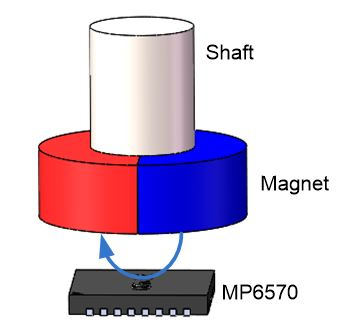Figure 1: How the MP6570 Senses Rotor Position

## GETTING THE ELECTRICAL ANGLE FROM THE SENSOR ANGLE

A 3-phase PMSM/BLDC motor is composed of two parts: the stator and the rotor.

The stator is composed of three windings coiled on the stator core. The windings of the three phases are separately placed by the rule of 120°. When an AC current passes through the three phase windings, a circular rotating magnet field (Fs) in space is generated, and the rotating frequency is the same as the AC current frequency.

The rotor is composed of one or more pairs of permanent magnetic poles to offer a constant rotor magnetic field (Fr).

In steady-state operation, Fr follows the stator magnetic Fs with the same rotating speed. If we stand on the axis of rotor field Fr to see the stator magnetic Fs, the stator magnetic Fs becomes a DC component. This means that we can find a proper coordinate transformation to switch the three-phase AC components to 2-phase DC components for easy control. The 2-phase DC components, called the d axis (rotor flux axis) component and q axis (leading d axis 90°) component, can be decoupled and controlled separately. This is called field-oriented control (FOC). Normally, the d axis current ID is zero, so that the stator flux is perpendicular with the rotor flux to achieve better efficiency and larger torque.

If the angle theta between the rotor flux d axis and Phase A axis is known, 3-to-2 transformation can be done easily (see Figure 2). The angle between the rotor flux and stator phase vector A is defined as the electrical angle.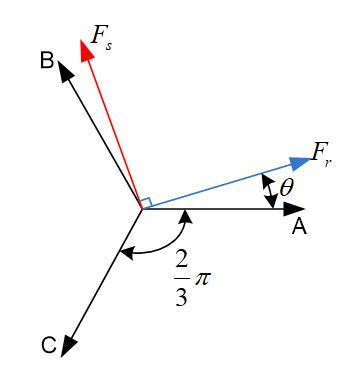Figure 2:Electrical Angle

During manufacturing, the magnet maybe mounted arbitrarily on the motor shaft with no fixed relationship between the rotor pole and magnet pole alignment. For different sets of motors, the angle obtained from the MP6570 angle sensor differs from the rotor electrical angle by some offset (see Figure 3).

## FIND THETA BIAS USING THE MP6570

When there is a positive current in motor winding A, the vector is shown as A+. When the current is negative, the vector is shown as A-. Figure 4 shows a space vector diagram with the six basic vectors.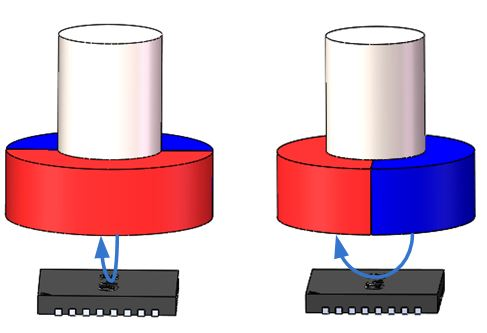Figure 3: Magnet Mounting on Different Motors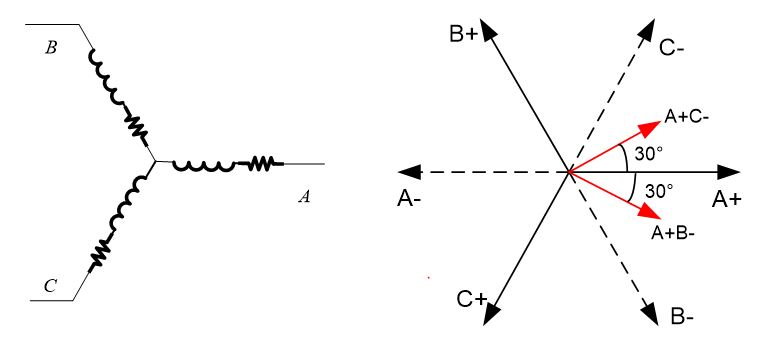Figure 4: Finding Theta Bias in a Star-Connected Motor

To get the position when the electrical angle is zero, a current should be applied to the motor winding A. However, in a real motor, there is no way to apply current to phase winding A only. The current must flow via either phase winding B or C. The vector sum of A+B- and A+C- is also aligned with vector A. Figure 5 shows how to get the initial theta bias with the MP6570.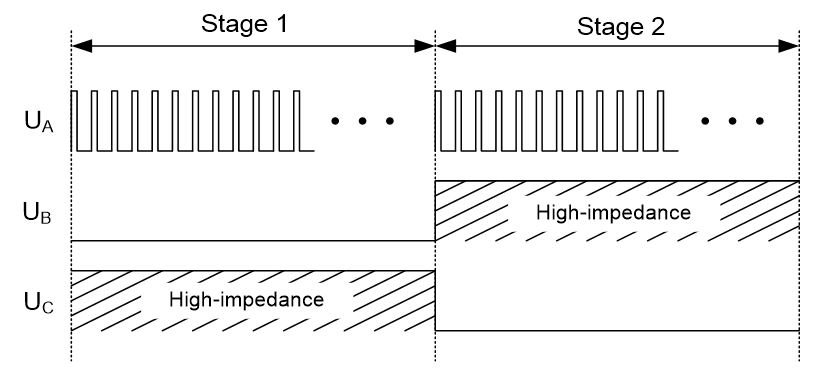Figure 5: Switching Logic during the Auto-Theta Bias Period

During stage 1, phase A is switching, the phase B low-side switch is on, and phase C is at high impedance. The rotor aligns to the A+B- vector position. During stage 2, phase A is switching, phase B is at high impedance, and the phase C low-side switch is on. The rotor is aligns to the A+C- vector position. By setting different phase A duty cycles and time intervals, the torque current aligning the rotor can be controlled to match the characteristics of the motor used. This operation can be done automatically by setting the duty and time interval to corresponding registers. This is can be done easily by following this guide or using the eMotion Virtual Bench, the GUI software developed by MPS.

## STAR AND DELTA CONNECTED MOTORS

The stator windings of three-phase BLDC/PMSM motors are made of three coils. There are two common electrical winding configurations: star and delta. The star configuration connects all of the windings to a central point, and power is applied to the remaining end of each winding (see Figure 6a).The delta configuration connects three windings to each other in a triangular circuit and power is applied at each of the connections (see Figure 6).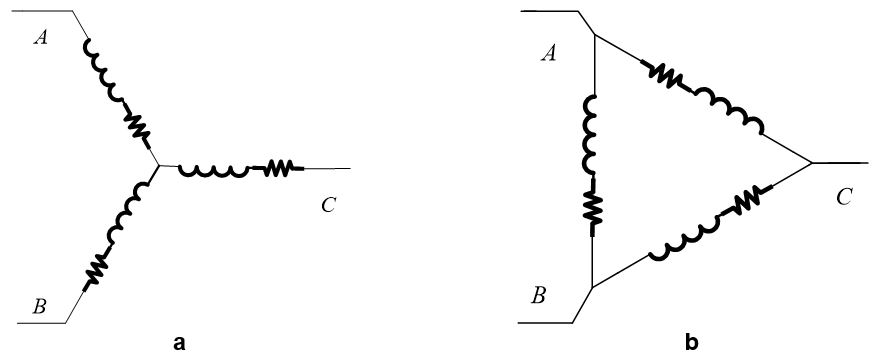Figure 6: Star and Delta Connection Circuit

The MP6570 can be used on both star- and delta-connected motors using the same aligning method to find the theta bias.

## DESIGN SUMMARY

When the rotor is aligning to Phase A, the sensor angle, called theta bias, should be found first to operate FOC control. This is can be done easily by following this guide or using the eMotion Virtual Bench.

The MP6570 can be used in a delta-connected motor. There is no need to change the theta bias.